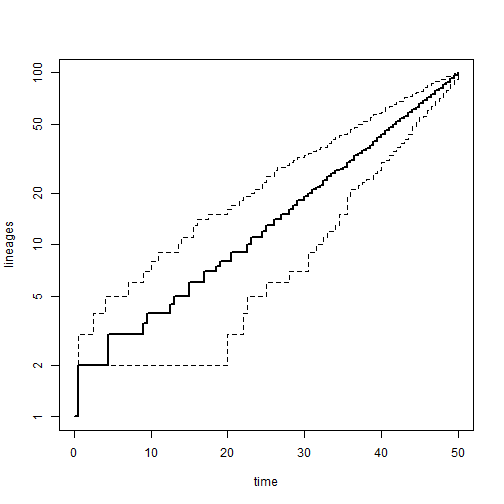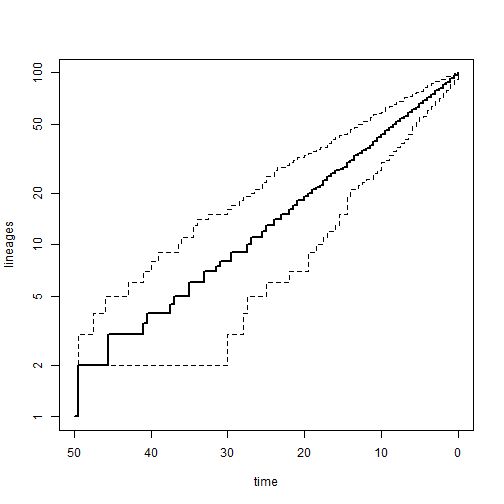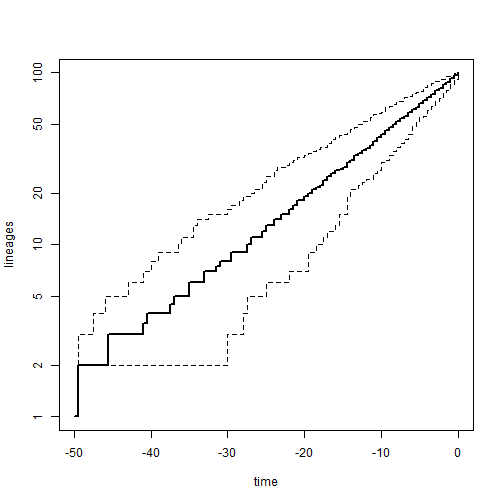## Saturday, October 25, 2014

### Reversing the time axis in an ltt95 plot

A phytools user recently asked the following:

“I am currently using the `ltt95` function on a `"multiPhylo"` object. I would like to known if there is a solution to reverse the time axis from the root of the tree to present?”

Indeed this is not too difficult. `ltt95` already returns an object of class `"ltt95"` invisibly. This object is basically a matrix containing the plotted data for x and y in columns, with a few different attributes to tell the S3 plotting method for objects of its class how to handle the matrix. So if we were naive as to the functionality of our plotting method, we could just manipulate the contents of that matrix to get the plot that we wanted. phytools makes things even easier, however, as there is an argument `xaxis` in the plotting method for objects of class `"ltt95"` that automates this all for us.

Here's how we can use it to control the direction of x in various ways:

``````## load phytools
library(phytools)
``````
``````## Loading required package: ape
``````
``````## simulate some trees
trees<-pbtree(n=100,scale=50,nsim=100)
## now create a standard ltt95 plot
obj<-ltt95(trees,log=TRUE)
````````````## OK - now if we want to simply flip the values on x
## so that they should "time before present"
plot(obj,xaxis="flipped")
````````````## we can also plot time before present as "negative time"
plot(obj,xaxis="negative")
``````Well, that's really it.